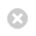Close

Mathematics

Home# Applied Analysis

The Applied Analysis group at UCL Mathematics has a broad range of research interests in asymptotic-, functional-, complex- and stochastic analysis.

Much of our research concerns the analysis of linear and
nonlinear ordinary and partial differential and difference equations.
Our work is motivated by applications in fluid dynamics, wave
propagation, geophysics, medical imaging, relativity theory, finance,
industry, and social science. Within the group we have particular expertise in:

• high frequency wave propagation and scattering;
• homogenization;
• hybrid numerical-asymptotic methods;
• integrable systems;
• integral equations and operator theory;
• inverse problems;
• kinetic theory;
• mathematical fluid mechanics;
• Nevanlinna theory;
• Sobolev spaces on non-smooth domains;
• spectral theory;
• stochastic PDEs.

Group members include: